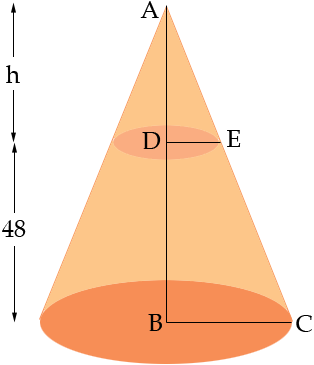SEARCH HOMEMath Central Quandaries & QueriesQuestion from jason: i need to know how to figure the cubic feet of a cone that the top is 72" wide the bottom is 25" wide and it is 48" tall. is there a specific formula for this?Hi Jason,

The shape you have is called a truncated cone and you can see the volume as the difference between the volumes of two cones.The volume of a cone is 1/3 π r2 h where r is the radius of the base, h is the height and π is approximately 3.1416. For your two cones the radius of the base of the larger cone is |BC| = 36 inches and the radius of the base of the larger cone is |DE| = 25/2 inches. The heights are h + 48 inches and h inches. Thus if you can find the length |AD| = h you can calculate the volumes.

The key to finding h is the triangles ADE and ABC. These triangles are similar so

(h + 48)/36 = h/(25/2)

Solving for h gives h = (48 × 25)/47 = 25.53 inches.

Use the expression for the volume of a cone to calculate the volumes of the two cones and then subtract these values to find the volume of the truncated cone.

I hope this helps,
PennyMath Central is supported by the University of Regina and The Pacific Institute for the Mathematical Sciences.